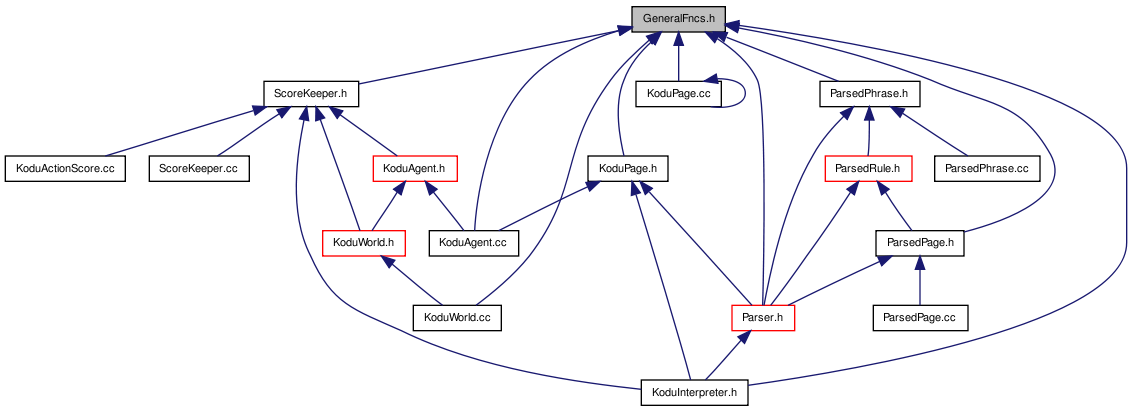# GeneralFncs.h File Reference

`#include <ctime>`
`#include <queue>`
`#include <vector>`
`#include <sys/time.h>`
Include dependency graph for GeneralFncs.h:This graph shows which files directly or indirectly include this file:Go to the source code of this file.

## Namespaces

namespace  GeneralFncs

## Functions

template<typename T >
void GeneralFncs::destroyAllPtrsInQueue (std::queue< T * > &q)
Deletes and erases each dynamically created element.
template<typename T >
void GeneralFncs::destroyAllPtrsInVector (std::vector< T * > &vec)
Deletes and erases each dynamically created element.
template<typename T >
void GeneralFncs::destroyPtrInVector (std::vector< T * > &vec, std::size_t elementIndex)
Deletes and erases a particular index in a vector.
template<typename T >
void GeneralFncs::deletePtr (T *&ptr)
Deletes the memory pointed by a pointer.
unsigned long GeneralFncs::getTime ()
Returns the time since in seconds (value has millisecond-precision).
template<typename T >
std::vector< T > GeneralFncs::subVector (const std::vector< T > &vec, const std::size_t startPos, const std::size_t endPos)
Returns a subset of a vector from the start position up to, but not including, the end position.

 Tekkotsu v5.1CVS Generated Mon May 9 04:58:56 2016 by Doxygen 1.6.3